# NOPAT

Net Operating Profit After Taxes

It stands for Net Operating Profit After Taxes, and it measures a company's profitability by ignoring the effect of debt on tax expenses. When companies take on debt, it implies tax-deductible interest expenses.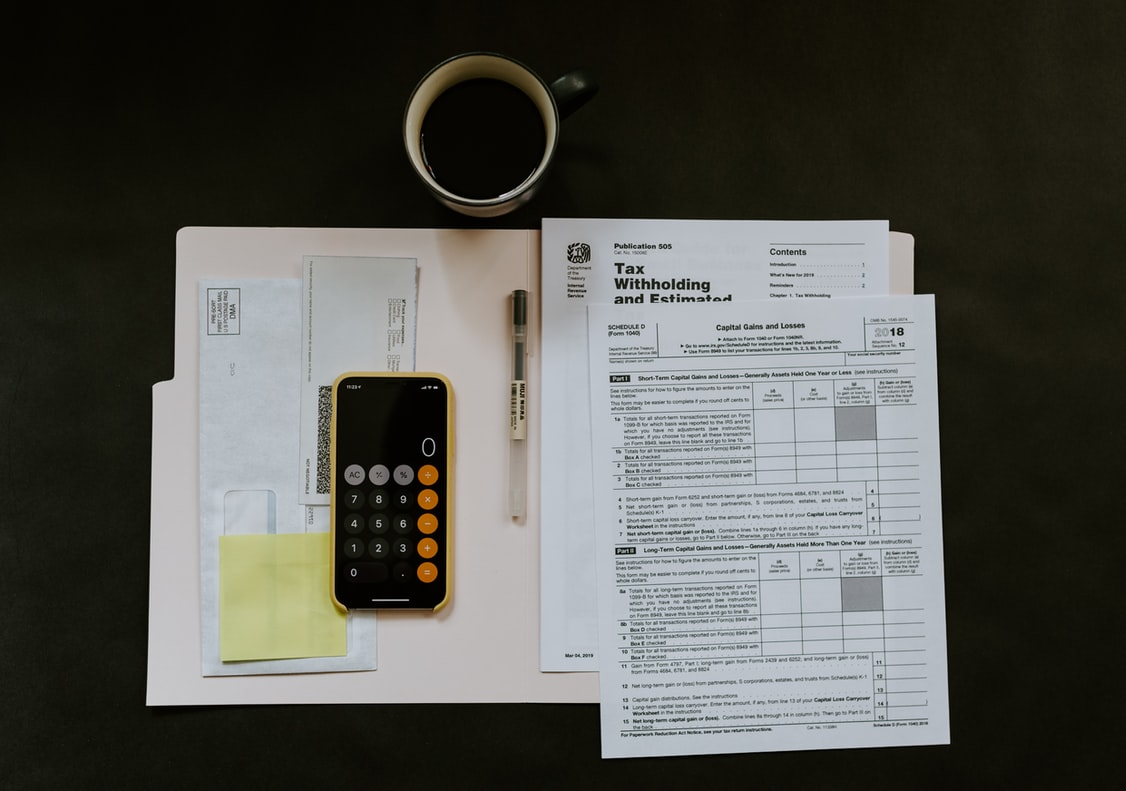Key points

•     It is based on the income statement.
•     Seeks to take off the tax benefit of debt.
•     It is the building block of unlevered cash flow.

To obtain a proxy for a company's cash generation, we must add back the interest expense while still considering the tax rate. That is what NOPAT is for.

We add back the interest expense because we are interested in assessing the potential of cash flow generation, and a company only pays interest if it has enough cash to do so. While taxes are paid whether the company has the cash or not.

The formula for is as follows: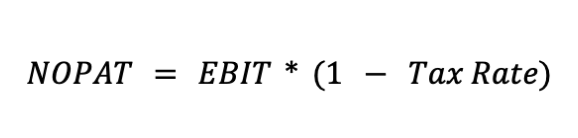As far as finance theory goes, we would want to consider the company's capital structure available to all stakeholders, not only the equity owners. That is why we add back the interest expense.

Now let's dive into each of these terms.

• EBIT

Stand for Earnings Before Interest and Taxes. It is used as a proxy for Operating Profit since the calculation behind each one is pretty much the same. It calculates NOPAT because it adds back the interest expense into the net income figure.

• Tax rate

The cash paid for taxes is divided by the EBT (Earnings Before Taxes) figure. Then, we multiply EBIT by 1 minus it to arrive at NOPAT.

How to calculate it?

Find the company's tax expense (in cash). A company usually discloses this in their income statement

1.  Identify the Earnings Before Taxes (EBT) amount
2. Divide the tax expense in cash by the EBT figure to arrive at the tax rate.
3. Find the EBIT figure.
EBIT maybe just understood as operating profit. For our calculation purposes, they are the same.
4. Now multiply the EBIT amount by one minus the tax rate, arriving at NOPAT.Example

Suppose Company A has an EBT figure of \$1,000 and a tax expense of \$200. We can quickly conclude that the tax rate is equal to 20%.

Following the steps outlined above, we must now find the EBIT amount. For example, suppose the company has an interest expense of \$ 300. By simply adding this expense to the Earnings Before Taxes, we find that EBIT equals \$ 1,300.

Now, we can find Net Operating Profit After Taxes.

By plugging these values into the formula, we obtain: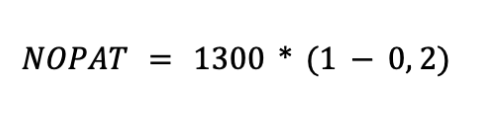Which yields: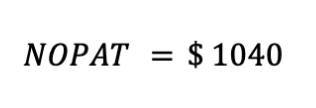That figure would represent the company's profitability if it were to pay taxes on all the money it earns, including the interest expenses. Notice that it is slightly greater than the EBT amount. This is because the larger the interest expense, the larger the disparity between EBT and NOPAT.

For instance, if we had 400 \$ as interest expense. EBIT would equal \$ 1400, and NOPAT would be 1120. That is the essence of this calculation. The more a company spends on servicing debt, the more cash it can generate to service it.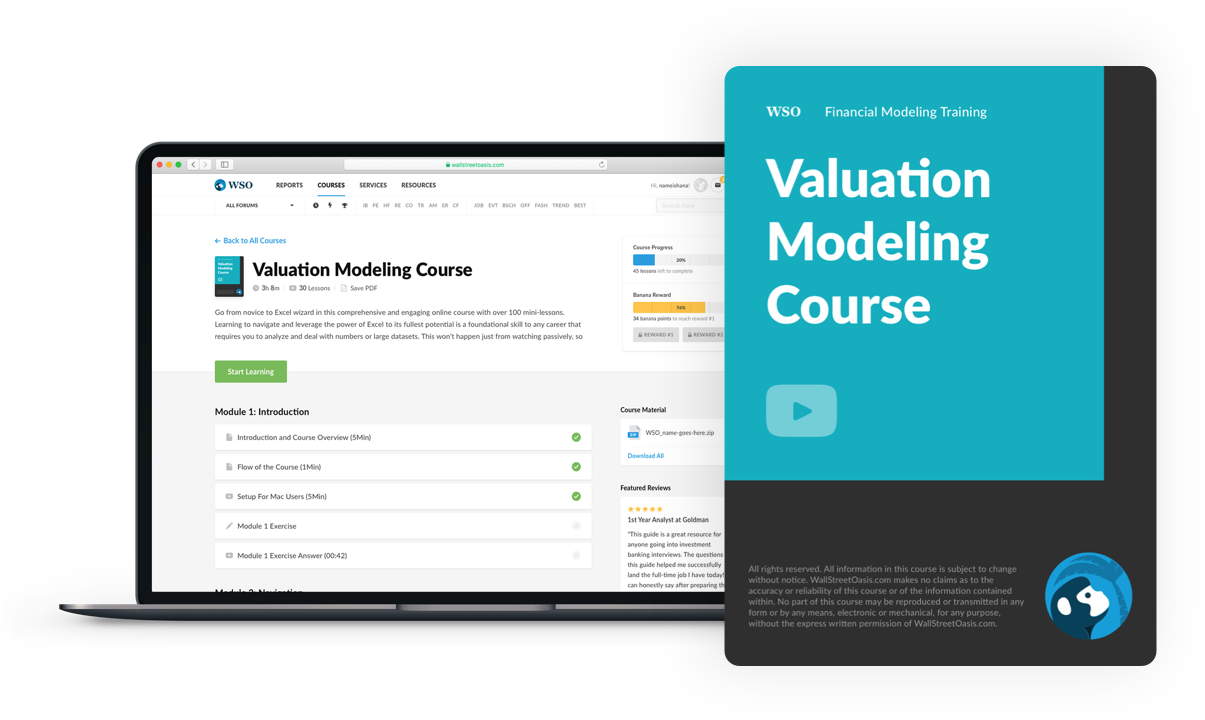### Everything You Need To Master Valuation Modeling

To Help You Thrive in the Most Prestigious Jobs on Wall Street.

## What is it used for?

NOPAT is used to derive Free Cash Flow to the Firm, which is a figure used in the Discounted Cash Flow to find the intrinsic value of companies.Now, let's break down each of these concepts.

• Free Cash Flow to the Firm

It is a cash flow metric. While NOPAT is taken from the income statement, which follows accrued accounting, FCFF is concerned with cash accounting, which doesn't consider proper revenue recognition rules. So instead, it accounts for each actual entry and exit of cash.

It adds back all non-cash expenses to NOPAT and discounts capital expenditures and changes in working capital.

• Discounted Cash Flow

Money today is worth more than money tomorrow. That is the rationale behind DCF

By taking a series of cash flows into the future and discounting them to present value, we arrive at a theoretical fair value for an asset.

The key assumption here is the discount rate, often taken as WACC.

• Valuation analysis

Valuation is a wide-ranging topic. However, it can be broken down into two main areas, intrinsic and comparable valuations. In intrinsic valuation, the main tool is the DCF. Since you are trying to grasp the value (measured in cash) the asset will generate.

Comparable valuation is performed as a quick method taking as a proxy the value of assets that are similar to the one you are trying to value.

For instance, if you are trying to evaluate a house, you'd seek similar houses in the same neighborhood, find out how much they are worth, and then arrive at a fair value for your target.

Now we can see why it is so important. NOPAT is the building block of valuation analysis. Since, ultimately, valuation is mainly concerned with cash flow generation, we must make cash adjustments to the base figure, leading us to FCFF.

## Diving deeper

To understand how it is used in practice, we must understand how a discounted cash flow analysis works.

It is a valuation method built upon the assumption that an asset's value is derived from its future cash flows. For that, we need a cash flow metric, and that is why we adjust Net Operating Profits After Taxes.To project these cash flows far into the future, we must put in place our assumptions that will set the path for our financial model

The number of assumptions will depend on the level of detail we want to achieve with our model. However, it should be enough to satisfy the variables we want to project.

The method by which we set up our assumptions can vary. For example, suppose it's an assumption related to a macroeconomic variable, such as GDP growth. In that case, the analyst will grab a survey and extract an average value.

On the other hand, if it is one related to the specifics of the company, it may be measured by digging into the company's filings and then drawing a conclusion.

Once we have the assumptions in place, we can project and link the financial statements with our financial model.

By forecasting the future earnings and then manipulating the tax rate and EBIT figures - provided we have good assumptions in place - we can then arrive at NOPAT.

Now, since it is a metric based on accrual accounting, and we are interested in cash flows, we must make adaptations to it.

1. Add back D&A expenses
We are adding back non-cash expenses, so we need to add back D&A.
2. Subtract capital expenditures
We subtract the Capex because we are interested in the cash flow that remains after the company's operational obligations are met.
3. Subtract working capital expenses

The same applies to working capital. Both capex and working capital are expenses that the company would not be able to survive without, which is the reason why it is subtracted.

Now we finally arrive at the unlevered cash flow. All we have to do now before performing the DCF is discount future cash flows to present value using an appropriate discount rate, which is usually the WACC.

The value we get by discounting it to present value is the Enterprise Value. However, if it is a public company we are evaluating, we are interested in the per-share value, so we must get to Equity Value. To do that, we subtract the net debt from the Enterprise Value

## Practical Example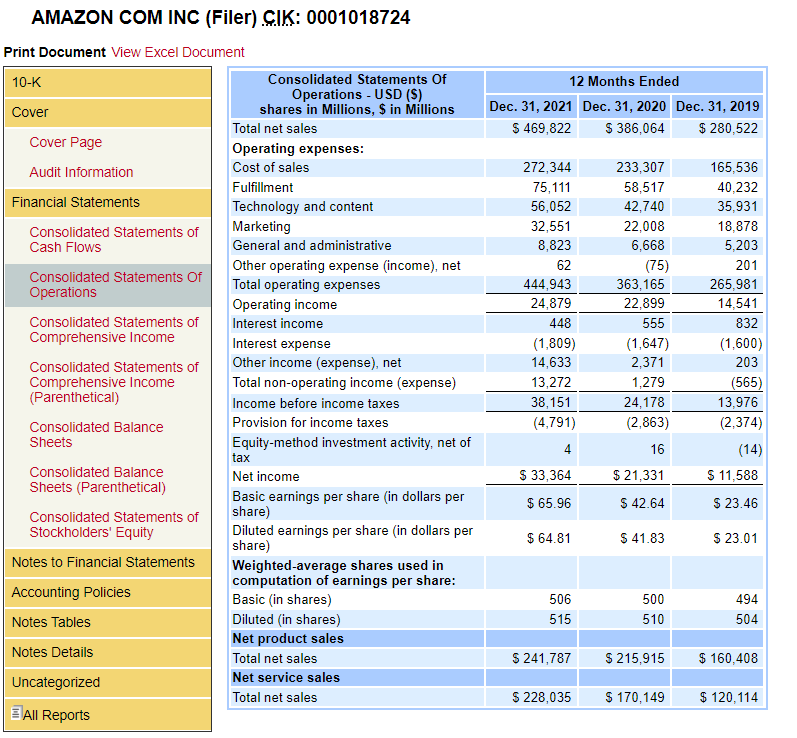In the above image, we have the latest 10-K filing from Amazon. Now let's derive its NOPAT.

We begin by finding the cash tax expense. We can see from the statements that the company laid out \$ 4,791 million in taxes.

Now let's find the earnings before taxes. In the row above, they outline the amount. EBT  comes to \$ 36,151 million.With both the tax expense and earnings before tax, we can find the effective tax rate for the business:

Tax rate = Tax amount/EBT = 4,791/36,151

Computing the calculation, we get a tax rate of 13.25%.

Now, finding the EBIT figure disclosed as operating income: 24,879 million \$.

After calculating both EBIT and tax rate, let us apply the formula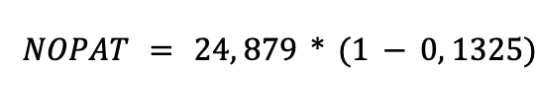In this case, comes out to be \$ 21,582 million.

However, it is essential to note that you would carry out this calculation in Excel in a much faster and more efficient way in real life. Therefore, it is important to brush up on your Excel skills before starting.

## FAQ

• What if the interest expense is not disclosed in the income statement?

Financial statements filed by public companies are required to feature explanatory notes, which is where you would find the details about whatever is listed on each of the statements.

• What is the difference between EBIT, EBT, and EBITDA?

EBIT stands for Earnings Before Interest and Taxes and is usually equal to operating profit. If it isn't explicitly laid out, you would add interest expense to the earnings before taxes.

EBT stands for Earnings Before Taxes. It is net income but before taxes. It is the amount upon which taxes are calculated.

EBITDA stands for Earnings Before Interest Taxes Depreciation & Amortization and equals EBIT plus D&A expenses. It is so commonly used because it adds back the main non-cash expense. It can serve as a proxy for cash flow

• I have arrived at NOPAT. Now how can I get to FCFF?

Once you have the metric in place, you have to follow the steps to arrive at free cash flow to the firm to perform the valuation analysis. However, finding accurate information such as changing working capital and capital expenditures may get tricky.

First of all, it is vital to understand the financial statements. You have to understand the capital expenditures profile of the company and the operational cash flow profile.

The idea is that by knowing how the company's financials work, you will be able to ignore non-recurring items, which must not be considered for the valuation analysis.

• What exactly are deferred taxes?

Taxes are tricky. The thing is that often the accounting schedule that companies follow does not match the schedule set out by the tax authorities, which can lead to mismatches. The company will incur either a tax asset or liability when that happens.

A tax asset is generated when the amount effectively paid is greater than the one derived from the tax rule. Conversely, the tax liability is generated when the amount effectively paid is less than the one derived from the tax rule.### Everything You Need To Master Excel Modeling

To Help You Thrive in the Most Prestigious Jobs on Wall Street.

Researched and authored by Lucas Amorim | LinkedIn

### Free Resources

To continue learning and advancing your career, check out these additional helpful WSO resources: